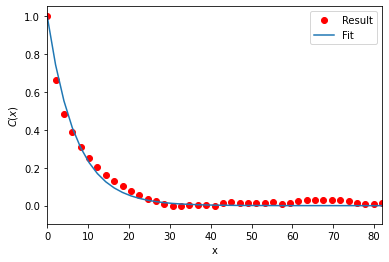# Determining the persistence length of a polymer

Here we determine the persistence length of a polymer.

Last updated: December 2022 with MDAnalysis 2.4.0-dev0

Minimum version of MDAnalysis: 1.0.0

Packages required:

:

import MDAnalysis as mda
from MDAnalysis.tests.datafiles import TRZ_psf, TRZ
from MDAnalysis.analysis import polymer
%matplotlib inline


The test files we will be working with here feature a pure polymer melt of a polyamide.

:

u = mda.Universe(TRZ_psf, TRZ)


## Choosing the chains and backbone atoms

We can define the chains of polyamide to be the common definition of a molecule: where each atom is bonded to at least one other in the group, and not bonded to any atom outside the group. MDAnalysis provides these as fragments.

:

chains = u.atoms.fragments


We then want to select only the backbone atoms for each chain, i.e. only the carbons and nitrogens.

:

backbones = [ch.select_atoms('not name O* H*') for ch in chains]


This should give us AtomGroups where the spatial arrangement is linear. However, the atoms are in index order. We can use sort_backbone to arrange our atom groups into their linear arrangement order.

:

sorted_bb = [polymer.sort_backbone(bb) for bb in backbones]


## Calculating the persistence length

The persistence length is the length at which two points on the polymer chain become decorrelated. This is determined by first measuring the autocorrelation $$C(n)$$ of two bond vectors $$(\mathbf{a}_i, \mathbf{a}_{i + n})$$ separated by $$n$$ bonds, where

$C(n) = \langle \cos\theta_{i, i+n} \rangle = \langle \mathbf{a_i} \cdot \mathbf{a_{i+n}} \rangle$

An exponential decay is then fitted to this, which yields the persistence length $$l_P$$ from the average bond length $$\bar{l_B}$$.

$C(n) \approx \exp\left( - \frac{n \bar{l_B}}{l_P} \right)$

We set up our PersistenceLength class (API docs). Note that every chain we pass into it must have the same length.

:

plen = polymer.PersistenceLength(sorted_bb)
plen.run()

:

<MDAnalysis.analysis.polymer.PersistenceLength at 0x7f4b7ddfd160>


The average bond length is found at plen.results.lb, the calculated persistence length at plen.results.lp, the measured autocorrelation at plen.results and the modelled decorrelation fit at plen.results.fit.

:

print(plen.results.fit.shape)
print('The persistence length is {:.3f}'.format(plen.results.lp))

(179,)
The persistence length is 6.917


MDAnalysis.analysis.polymer.PersistenceLength provides a convenience method to plot the results.

:

plen.plot()

:

<AxesSubplot:xlabel='x', ylabel='$C(x)$'>